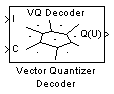Vector Quantizer Decoder

Find vector quantizer codeword that corresponds to given, zero-based index value

Library

Quantizers

dspquant2

•Description

The Vector Quantizer Decoder block associates each input index value with a codeword, a column vector of quantized output values defined in the Codebook values parameter. When you input multiple index values into this block, the block outputs a matrix of quantized output vectors. This matrix is created by horizontally concatenating the codeword vectors that correspond to each index value.

You can select how you want to enter the codebook values using the Source of codebook parameter. When you select Specify via dialog, you can type the codebook values into the block parameters dialog box. Select Input port and port C appears on the block. The block uses the input to port C as the Codebook values parameter.

The Codebook values parameter is a k-by-N matrix of values, where k ≥ 1 and N ≥ 1. Each column of this matrix is a codeword vector, and each codeword vector corresponds to an index value. The index values are zero based; therefore, the first codeword vector corresponds to an index value of 0, the second codeword vector corresponds to an index value of 1, and so on.

The input to this block is a vector of index values, where 0 ≤ index < N and N is the number of columns of the codebook matrix. Use the Action for out of range index value parameter to determine how the block behaves when an input index value is out of this range. When you want any index values less than 0 to be set to 0 and any index values greater than or equal to N to be set to N-1, select Clip. When you want to be warned when any index values less than 0 are set to 0 and any index values greater than or equal to N are set to N-1, select Clip and warn. When you want the simulation to stop and display an error when the index values are out of range, select Error.

Data Type Support

The input to the block can be the index values and the codebook values. The data type of the index input to the block at port I can be uint8, uint16, uint32, int8, int16, or int32. The data type of the codebook values can be double, single, or Fixed-point.

The output of the block is the quantized output values. These quantized output values always have the same data type as the codebook values. When the codebook values are specified via an input port, the block assigns the same data type to the Q(U) output port. When the codebook values are specified via the dialog, use the Codebook and output data type parameter to specify the data type of the Q(U) output port. The data type of the codebook and quantized output can be Same as input, double, single, Fixed-point, User-defined, or Inherit via back propagation.

Dialog Box

The Main pane of the Vector Quantizer Decoder block dialog appears as follows.Action for out of range index value

Choose the behavior of the block when an input index value is out of range, where 0 ≤ index < N and N is the length of the codebook vector. Select Clip when you want any index values less than 0 to be set to 0 and any index values greater than or equal to N to be set to N-1. Select Clip and warn when you want to be warned when any index values less than 0 are set to 0 and any index values greater than or equal to N are set to N-1. Select Error when you want the simulation to stop and display an error when the index values are out of range.

Source of codebook

Choose Specify via dialog to type the codebook values into the block parameters dialog box. Select Input port to specify the codebook values using the block's input port, C.

Codebook values

Enter a k-by-N matrix of quantized output values, where 1 ≤ k and 1 ≤ N . Each column of your matrix corresponds to an index value. This parameter is visible if, from the Source of codebook list, you select Specify via dialog.

The Data Types pane of the Vector Quantizer Decoder block dialog appears as follows.Codebook and output data type

Specify the data type of the codebook and quantized output values. You can select one of the following:

• A rule that inherits a data type, for example, Inherit: Same as input.

• A built in data type, such as double

• An expression that evaluates to a valid data type, for example, fixdt(1,16)

Click the buttonto display the Data Type Assistant, which helps you set the Output data type parameter.

This parameter is available only when you set the Source of codebook parameter to Specify via dialog. If you set the Source of codebook parameter to Input port, the output values have the same data type as the input codebook values.

References

Gersho, A. and R. Gray. Vector Quantization and Signal Compression. Boston: Kluwer Academic Publishers, 1992.

Supported Data Types

PortSupported Data Types

I

• 8-, 16-, and 32-bit signed integers

• 8-, 16-, and 32-bit unsigned integers

C

• Double-precision floating point

• Single-precision floating point

• Fixed point

• 8-, 16-, and 32-bit signed integers

Q(U)

• Double-precision floating point

• Single-precision floating point

• Fixed point

• 8-, 16-, and 32-bit signed integers

• 8-, 16-, and 32-bit unsigned integers

 Quantizer (Simulink) Simulink Scalar Quantizer Decoder DSP System Toolbox Uniform Encoder DSP System Toolbox Uniform Decoder DSP System Toolbox Vector Quantizer Encoder DSP System Toolbox

Extended Capabilities

Fixed-Point ConversionDesign and simulate fixed-point systems using Fixed-Point Designer™.

Introduced before R2006a

DSP System Toolbox DocumentationGet trial now